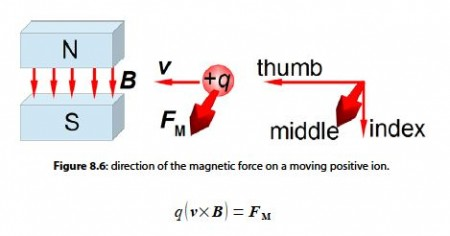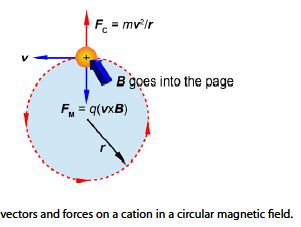# Magnetic Sector Mass Spectrometer

A cation of charge q travelling with a velocity v enters a magnetic field B at right angles (θ = 90°) to the field and experiences a magnetic force FM which is the vector product, below. Draw a diagram to describe the direction of the force felt by a cation. What would be the direction of the force vector for an anion?

q (v x B)= FM

In a practical mass spectrometer the magnetic sector is not straight but the north and south poles are both curved round into an arc of a circle (typically 90°). The ion follows a circular path of radius r whilst inside this field. The centripetal force FC required to balance this magnetic force is FC = mv2 / r.

mv2 / r = q (v x B

Draw a vector diagram of the various vectors and forces experienced by the positive ions during its time inside the circular magnetic field.

SolutionFor a negative ion the force vector would point in the opposite direction.